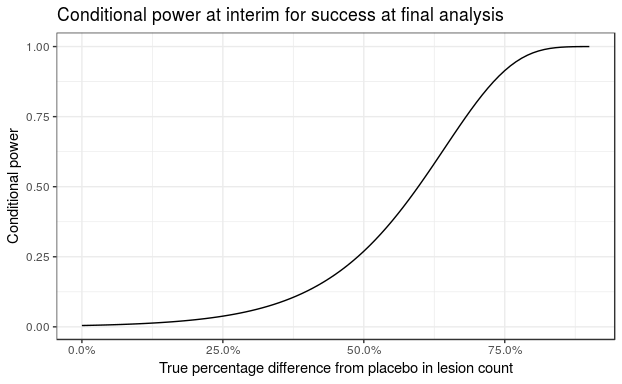# Probability of Success at an Interim Analysis

#### 2019-04-04

At different stages of drug development, there are questions about how likely a study will be successful given previously collected data within the trial itself or data from other earlier trials. For example given Ph2a (PoC, proof of concept) and Ph2b (DRF, dose range finding) studies, how likely a new Ph3 study would be successful is of great interest. Another example, at an interim analysis (IA) of a PoC study, one would also be interested to understand the probability of success at the end of the study given the partial data observed. pos1S() and pos2S() are constructed to calculate predictive probabilities for this purpose to inform quantitative decision making. This vignette shows an example from an IA in a PoC study, where pos2S() was used to explore the probability of success for the final analysis given the interim data.

The primary endpoint for this study is log transformed facial lesion count, assumed to be normally distributed. Decrease in the lesion count upon treatment is considered improvement in the patients. Below is the summary statistics of this primary endpoint by group at the interim.

ia <- data.frame(n=c(12, 14),
median_count=c(20.5, 21),
mean_count=c(23.3, 27),
mean_log=c(2.96, 3.03),
sd_log=c(0.67, 0.774),
row.names=c("active", "placebo")) %>%
transform(se_log=round(sd_log/sqrt(n), 3))
sd_log_pooled <- with(ia, sqrt(sum(sd_log^2*(n-1))/(sum(n)-2)))
kable(ia)
n median_count mean_count mean_log sd_log se_log
active 12 20.5 23.3 2.96 0.670 0.193
placebo 14 21.0 27.0 3.03 0.774 0.207

The predefined dual PoC criteria is as follows,

n <- 21 # planned total n per arm
rules <- decision2S(c(0.9, 0.5), c(0,-0.357), lower.tail = TRUE)
print(rules)
## 2 sample decision function
## Conditions for acceptance:
## P(x1 - x2 <= 0) > 0.9
## P(x1 - x2 <= -0.357) > 0.5
## Link: identity

The interim data were evaluated against the PoC criteria with weakly informative priors for both active and placebo groups. The criteria were not met, although it seemed to show some benefit of the active treatment over placebo numerically. The variability of this endpoint is higher than what was assumed for study sample size calculation.

priorP <- priorT <- mixnorm(c(1, log(20), 1), sigma = 0.47, param = 'mn')
## posterior at IA data
postT_interim <- postmix(priorT, m=ia["active","mean_log"], se=ia["active","se_log"])
postP_interim <- postmix(priorP, m=ia["placebo","mean_log"], se=ia["placebo","se_log"])
pmixdiff(postT_interim, postP_interim, 0)
##  0.5900663
pmixdiff(postT_interim, postP_interim,-0.357)
##  0.12637

The probability of success at the final analysis, i.e. the probability of meeting PoC criteria at trial completion given observed interim data, was computed using function pos2S(). One could assume that the new data after the interim would be from the same distribution as the interim data. If the $$\sigma_{1}$$ and $$\sigma_{2}$$ in pos2S() were not specified, i.e. the previously assumed $$\sigma$$ would be used.

pos_final <- pos2S(
postT_interim,
postP_interim,
n - ia["active","n"],
n - ia["placebo","n"],
rules,
sigma1 = sd_log_pooled,
sigma2 = sd_log_pooled
)

The function constructed by pos2S() can produce the predictive probability given any defined distribution for the two groups. For example, if the interim posterior distributions are used, the calculated probability is small, suggesting a low chance of success at the final analysis given observed IA data.

pos_final(postT_interim, postP_interim)
##  0.02413245

One can also use oc2S() to compute conditional power for any given treatment effect.

ia_oc <- oc2S(
postT_interim,
postP_interim,
n - ia["active","n"],
n - ia["placebo","n"],
rules,
sigma1 = sd_log_pooled,
sigma2 = sd_log_pooled
)

delta <- seq(0, 0.9, 0.01) #pct diff from pbo
pbomean <- ia["placebo","mean_log"]
y1 <- log(exp(pbomean) * (1 - delta)) #active
y2 <- log(exp(pbomean) * (1 - 0 * delta)) #placebo

out <-
data.frame(
diff_pct = delta,
diff = round(y1 - y2, 2),
y_act = y1,
y_pbo = y2,
cp = ia_oc(y1, y2)
)

ggplot(data = out, aes(x = diff_pct, y = cp)) + geom_line() +
scale_x_continuous(labels = scales::percent) +
labs(y = 'Conditional power',
x = 'True percentage difference from placebo in lesion count',
title = 'Conditional power at interim for success at final analysis')### R Session Info

## R version 3.5.1 (2018-07-02)
## Platform: x86_64-pc-linux-gnu (64-bit)
## Running under: Ubuntu 16.04.5 LTS
##
## Matrix products: default
## BLAS: /usr/lib/libblas/libblas.so.3.6.0
## LAPACK: /usr/lib/lapack/liblapack.so.3.6.0
##
## locale:
##  C
##
## attached base packages:
##  stats     graphics  grDevices utils     datasets  methods   base
##
## other attached packages:
##  scales_1.0.0    bindrcpp_0.2.2  ggplot2_3.1.0   purrr_0.2.5
##  dplyr_0.7.8     bayesplot_1.6.0 knitr_1.21      RBesT_1.3-8
##  Rcpp_1.0.0
##
## loaded via a namespace (and not attached):
##   rstan_2.18.2         tidyselect_0.2.5     xfun_0.4
##   reshape2_1.4.3       colorspace_1.3-2     htmltools_0.3.6
##   stats4_3.5.1         loo_2.0.0            yaml_2.2.0
##  rlang_0.3.0.1        pkgbuild_1.0.2       pillar_1.3.0
##  glue_1.3.0           withr_2.1.2          matrixStats_0.54.0
##  bindr_0.1.1          plyr_1.8.4           stringr_1.3.1
##  munsell_0.5.0        gtable_0.2.0         mvtnorm_1.0-8
##  codetools_0.2-15     evaluate_0.12        labeling_0.3
##  inline_0.3.15        callr_3.1.0          ps_1.2.1
##  parallel_3.5.1       highr_0.7            backports_1.1.3
##  rmarkdown_1.11       R6_2.3.0             compiler_3.5.1